# Parts of a Wave

We will be considering the parts of a wave with the wave represented as a transverse wave as in the following diagram:In the above diagram the white line represents the position of the medium when no wave is present. This medium could be imagined as a rope fixed at one end a few feet above the ground and held by you at the other end.

The yellow line represents the position of the medium as a wave travels through it. We simply say that the yellow line is the wave. If we consider the rope mentioned before, this wave could be created by vertically shaking the end of the rope.

Often, when several waves are traveling along a medium as shown above, the continuous group of waves is called a wave train. And, since the waves are made again and again, with each wave requiring the same amount of time for its creation, we say that the wave is periodic.

The section of the wave that rises above the undisturbed position is called the crest. That section which lies below the undisturbed position is called the trough. These sections are labeled in the following diagram: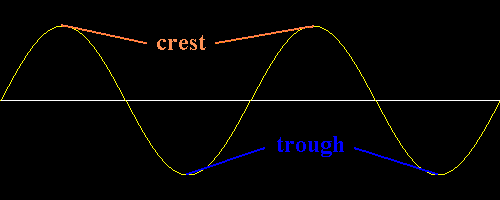The term amplitude can have slightly different meanings depending upon the context of the situation.

Its most general definition is that the amplitude is the maximum positive displacement from the undisturbed position of the medium to the top of a crest. This is shown in the following diagram:In some discussions it is important to distinguish between positive and negative amplitudes. These displacements are shown in the following diagram: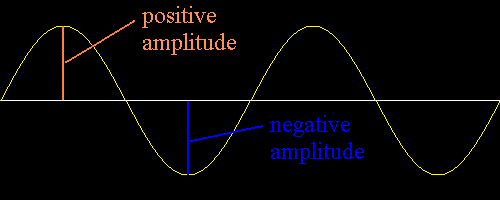Sometimes it is necessary to discuss an amplitude at a certain point along the wave. Several of these amplitudes are shown in the following diagram: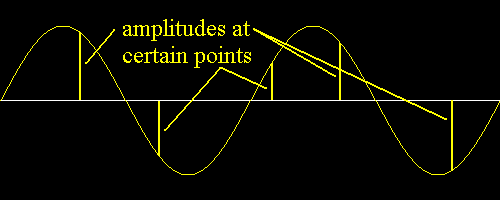Notice in the above diagram that three of the amplitudes are positive and two are negative.

Here are some other labeled examples of positive and negative amplitudes: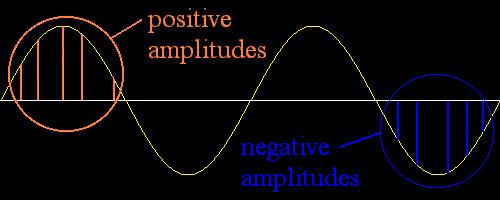In general, if the question simply is 'What is the amplitude of the wave?', the answer follows the description of amplitude shown in the first of the above four amplitude diagrams. It is the maximum positive displacement of the medium from its undisturbed position to the top of a crest.

In many discussions, though, the term amplitude takes on a slightly more complicated meaning. For example, in a discussion about wave interference the later descriptions of positive and negative amplitudes at certain points would be used. In such contexts, amplitude means the displacement of the medium from its undisturbed position to its disturbed position at a certain point along the wave.

All of this becomes clear as you study waves further and understand the context of your situation.

To sum up amplitude, we would say:

• It is the displacement of the medium from its normal position.
• Usually this simply means the maximum positive displacement.
• Often, especially in discussions about interference, amplitude means the displacement of the medium from its normal position at certain points, and this displacement can be positive or negative.

The wavelength of a wave is the distance between any two adjacent corresponding locations on the wave train. This distance is usually measured in one of three ways: crest to next crest, trough to next trough, or from the start of a wave cycle to the next starting point. This is shown in the following diagram:Actually, the a wavelength exists between any point on a wave and the corresponding point on the next wave in the wave train. A few of such distances are shown below: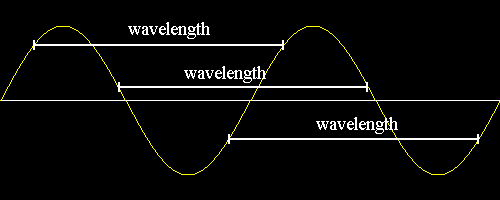Frequency is often not termed as a part of a wave, but it makes sense to introduce its meaning in this section.

Frequency refers to how many waves are made per time interval. This is usually described as how many waves are made per second, or as cycles per second.

The following interactive diagram lets you adjust the frequency of the wave train.

Frequency:

WARNING: For most waves thought about in physics, like sound waves or light waves, when you change the frequency, you also change the wavelength. That makes the velocity constant, which is true for sound and light waves. The above animation is not meant to show what happens specifically with sound and light waves. The animation is about waves in general, and what is meant by frequency. It is meant to show what happens when only the frequency changes, and not show the corresponding change in wavelength that happens with sound and light waves.

If ten waves are made per second, then the frequency is said to be ten cycles per second, written as 10 cps.

Usually, we use the unit Hertz to state frequency. A frequency of 10 cps is noted as a frequency of 10 Hertz. So, one cycle per second is one Hertz, as in:

1 cps = 1 Hertz

The unit Hertz is abbreviated this way:

1 Hertz = 1 Hz

Custom Search